Courses
Courses for Kids
Free study material
Free LIVE classes
More

# What is Subtraction?## Introduction to Subtraction

Learning subtraction is different than learning addition, but kids can perform it effortlessly if taught correctly- start with simple numbers and go slowly. You can progress to more advanced subtraction sums when you develop a solid foundation. You can accomplish this by explaining subtraction as taking away, using number lines, and practising subtraction vocabulary.

In Mathematics, subtraction is the removal of items from a group. Always remember that we start subtraction from one’s place. Using the number line, we can subtract the integers. You can add a positive integer by going to the right on the number line. Subtraction is when we remove a specified number of objects from a specified collection of objects. The '' symbol represents it. When one number is subtracted from another, the result is known as the difference.

## How to Borrow in Subtraction?

When subtracting big integers, the top digit in a column may be less than the bottom digit in that column. In that situation, you must borrow from the column to your left.

subtraction with borrowing consists of two steps:

• Cross out the number from which you're borrowing, subtract one, and write the result above the number you crossed out.

• Add 10 to the number at the top of the column you were working in.

For example: In subtracting 26 from 53, as you can notice, the upper rightmost digit of the first number (i.e. 3) is smaller than the lower number’s digit (i.e. 6). Since 3 < 6, we borrow from the left side which is 5 and add 10 to that number the 3 becomes

(10 + 3) = 13. Subtract one from the top number in the column on the left (i.e. 5 1 = 4).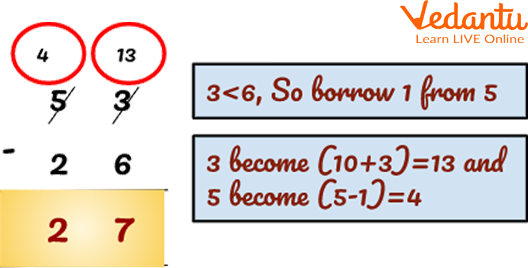Subtraction with Borrowing

## Subtraction with Carrying Over

Let's say you wish to subtract 34 from 926.

• The first step is to remove 4 from 6 in the ones column, yielding 2.

• When you move to the tens column 3, however, you need to subtract 2 – 3. Because 2 is smaller than 3, you need to borrow from the hundreds column.

• First, cross out the 9 and replace it with 8, because 9 – 1 = 8. Next, add 10 into 2, changing it to 12, because 2 + 10 = 12. Now you can subtract in the tens column: 12 – 3= 9.

• The final step is simple: 8 – 0 = 8.

• Hence, the answer is 892.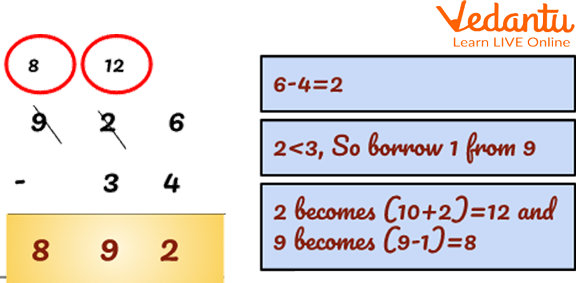Subtraction with Carrying Over

Let takes another example of subtraction borrowing problems:

Q.1 Subtract 458 67.

Ans: The steps to solve this problem is as follows:

1. The first step is to remove 7 from 8 in the ones column, yielding 1.

2. When you move to the tens column 5, however, you need to subtract 5 – 6. Because 5 is smaller than 6, you need to borrow from the hundreds column.

3. First, cross out the 4 and replace it with a 3, because 4 – 1 = 3.

4. Next, place a 1 in front of the 5, changing it to 15, because 5 + 10 = 15. Now you can subtract in the tens column: 15 – 6= 9.

5. The final step is simple: 3– 0 = 3.

6. Hence, the answer is 391.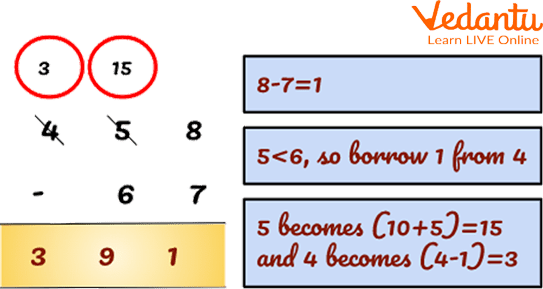Subtraction with Carrying Over

Q 2. Subtract 245 36

Ans: The steps to solve this problem is as follows:

1. Firstly, we can borrow 10 from tens place to one’s place.

2. Now, begin the subtraction.

3. In ones place, subtract 6 from 15 i.e. 15 – 6 = 9.

4. In tens place, after lending 10 to ones place, it is left with 3. Now, subtract 3 from 3 that is 3 – 3 = 0.

5. In the hundredth place, 2 is left which is written as it is.

6. Hence 245 - 36 = 209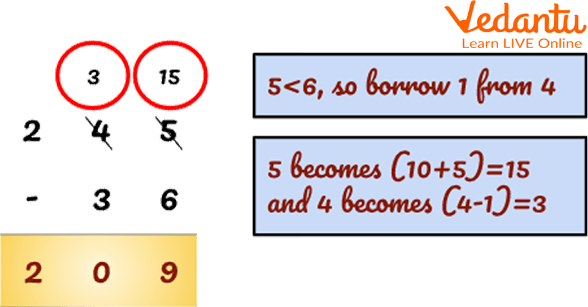Subtraction of 245 36

Q 3. Subtract 952 456.

Ans: The steps to solve this problem is as follows:

1. First, borrow 10 from tens places to one's places.

2. Now, begin the subtraction.

3. In ones place, subtract 6 from 12. I.e. 12 6 = 6.

4. In tens place, after lending 10 to ones place it is left with 4. Now borrow 10 from hundreds of places.

5. In tens place subtract 5 from 14 i.e. 14 5 = 9.

6. After lending to tens place it is left with 8.

7. In the hundreds place, subtract 8 4 = 4

Hence, 952 456 = 496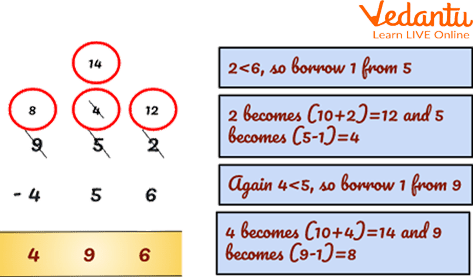Subtraction of 952 456

Q 1. Subtract 933 456.

Ans: 477

Q 2. Subtract 10000 12.

Ans: 9988

## Summary

In this article, we have discussed that subtraction is done or used when we are asked to remove a specified number of objects from a specified collection of objects. Always start subtracting from one’s place. Subtraction can be done by borrowing or without borrowing. Subtraction with borrow is used when the right-most digit of the first number is smaller than the right-most digit of the second number. Always start subtracting from one’s place.

Last updated date: 16th Sep 2023
Total views: 122.4k
Views today: 1.22k

## FAQs on What is Subtraction?

1. Is borrowing and regrouping in subtraction the same thing?

Subtraction with regrouping aids in the understanding and executing subtractions by utilising the concept of regrouping, also known as carrying or borrowing. Subtraction with regrouping is possible if the place value is kept in mind. The subtraction formula is written as Minuend - Subtrahend = Difference. Regrouping in subtraction is also known as carrying and borrowing. It is the process of creating groups of tens when adding or subtracting two-digit numbers (or more than two).

2. What separates subtraction from subtraction using borrow instruction?

Subtraction with regrouping is the process of vertically arranging two or more large numbers to find the difference between two given numbers. When adding or subtracting two-digit numbers (or more), regrouping is the process of forming groups of tens, which is also known as carrying and borrowing. Borrowing occurs more frequently in subtraction with regrouping, where we subtract one number from the corresponding place value. When the bottom number is greater than the top number, subtraction with borrowing is used. When subtracting a number that is greater than another in subtraction we use borrow. No borrowing or regrouping would be required for 35 - 3. In this case, 35 - 8 would require borrowing as 5 cannot be subtracted from 8.